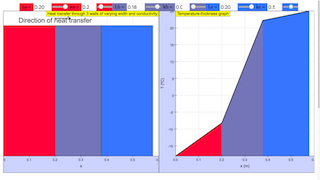Description

Multi-layered wall
The goal of this virtual lab is to illustrate the computation of the steady-state heat conduction in a three-layered wall. The steady-state heat conduction is assumed to be one-dimensional.

The wall is composed of three layers of different materials and thickness LA, L B an LC . The temperature of the inner surface of the wall is T1 and the outside temperature T4 (see figure 1).
The heat flow per unit area (qx )and the temperature at the interfaces of the diferent layers (T 2, T3 ) are calculated.
Thermal conductivities of the layer's materials (kA , kB , kC ) are assumed to be independent of others model variables. The thickness of the layer that compose the walls are model parameters. The wall surface (S) is assumed to be constant. The S parameter is not used in the model equations, because qx doesn't depend on this parameter.

The mathematical model is described by the following equations:
T1 - T2 = (La/ka)  *  qx T2 - T3 = (Lb/kb)  *  qx T3 - T4 = (Lc/kc)  *  qx
Authors Carla Martín
Dpto. de Informática y Automática
E.T.S. de Ingeniería Informática, UNED
Juan del Rosal 16, 28040 Madrid, Spain

### Translations

Code Language Translator Run### Software Requirements

SoftwareRequirements

 Android iOS Windows MacOS with best with Chrome Chrome Chrome Chrome support full-screen? Yes. Chrome/Opera No. Firefox/ Samsung Internet Not yet Yes Yes cannot work on some mobile browser that don't understand JavaScript such as..... cannot work on Internet Explorer 9 and below

### CreditsCarla Martn; Tan Wei Chiong; Loo Kang Wee

[text]

### For Teachers

Heat conducts through solids at different rates, and is dependent on the thickness of the solid and the heat conductivity of the solid.

We call this a steady-state conduction if the temperature difference driving the heat transfer is constant. In this simulation, it is assumed that the left side of a three-layered solid is set at a constant 25°C and the right side is set at a constant 0°C.

This simulation assumes that there is no loss of heat from the system (i.e.: this is a closed system). There are 3 walls in the graph on the left, representing 3 different solids that the heat conducts through. The graph on the right plots the temperature-thickness graph, showing how the temperature changes through the solids, and color-coded to represent the solid the heat travels through at that point.

There are 6 sliders to adjust the thickness and conductivity of each wall at the top of the simulation.

Research

[text]

[text]

[text]